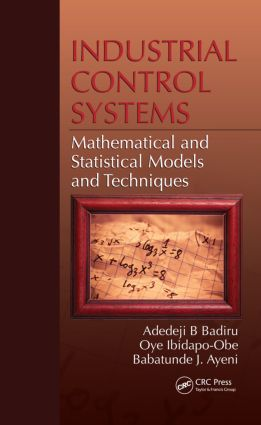# Industrial Control Systems

## Mathematical and Statistical Models and Techniques, 1st Edition

CRC Press

382 pages | 131 B/W Illus.

Hardback: 9781420075588
pub: 2011-10-24
SAVE ~\$22.00
\$110.00
\$88.00
x
eBook (VitalSource) : 9780429139871
pub: 2016-04-19
from \$55.00

FREE Standard Shipping!

### Description

Issues such as logistics, the coordination of different teams, and automatic control of machinery become more difficult when dealing with large, complex projects. Yet all these activities have common elements and can be represented by mathematics. Linking theory to practice, Industrial Control Systems: Mathematical and Statistical Models and Techniques presents the mathematical foundation for building and implementing industrial control systems. The book contains mathematically rigorous models and techniques generally applicable to control systems with specific orientation toward industrial systems.

An amalgamation of theoretical developments, applied formulations, implementation processes, and statistical control, the book covers:

• Industrial innovations and systems analysis
• Systems fundamentals
• Technical systems
• Production systems
• Systems filtering theory
• Systems control
• Linear and nonlinear systems
• Switching in systems
• Systems communication
• Transfer systems
• Statistical experimental design models (factorial design and fractional factorial design)
• Response surface models (central composite design and Box–Behnken design)

Examining system fundamentals and advanced topics, the book includes examples that demonstrate how to use the statistical designs to develop feedback controllers and minimum variance controller designs for industrial applications. Clearly detailing concepts and step-by-step procedures, it matches mathematics with practical applications, giving you the tools to achieve system control goals.

Mathematical Modeling for Product Design

Introduction

Literature Review

Memetic Algorithm and Its Application to Collaborative Design

Conclusion

References

Dynamic Fuzzy Systems Modeling

Introduction: Decision Support Systems, Uncertainties

Decision Support Systems

Uncertainty

Fuzziness

Fuzzy Set Specifications

Stochastic–Fuzzy Models

Applications

Conclusions

References

Stochastic Systems Modeling

Introduction to Model Types

Systems Filtering and Estimation

Correlation Techniques

Model Control—Model Reduction, Model Analysis

References

Systems Optimization Techniques

Optimality Conditions

Basic Structure of Local Methods

Stochastic Central Problems

Intelligent Heuristic Models

Heuristics

References

Statistical Control Techniques

Statistical Process Control

Control Charts

Process Capability Analysis

Time Series Analysis and Process Estimation

Time Series Analysis Example

Exponentially Weighted Moving Average

Cumulative Sum Chart

Statistical Process Control

Automatic Process Control

Criticisms of SPC And APC

Overcompensation, Disturbance Removal, and Information Concealing

Integration Of SPC And APC

ARIMA Modeling Of Process Data

Model Identification And Estimation

Minimum Variance Control

Process Dynamics With Disturbance

References

Design of Experiment Techniques

Factorial Designs

Factorial Design for 3 Factors

Saturated Designs

Central Composite Designs

Response Surface Optimization

References

Risk Analysis and Estimation Techniques

Bayesian Estimation Procedure

Computational Procedure

Parameter Estimation for Hyperbolic Decline Curve

Robustness of Decline Curves

Mathematical Analysis

Statistical Analysis

Parameter Estimation

Optimization Technique

Iterative Procedure

Residual Analysis Test

Simplified Solution to the Vector Equation

Integrating Neural Networks And Statistics For Process Control4

Fundamentals of Neural Network

The Input Function

Transfer Functions

Statistics and Neural Networks Predictions

Statistical Error Analysis

Integration of Statistics And Neural Networks

References

Mathematical Modeling and Control of Multi- Constrained Projects

Introduction

Literature Review

Methodology

Representation of Resource Interdependencies and Multifunctionality

Modeling of Resource Characteristics

Resource Mapper

Activity Scheduler

Model Implementation And Graphical Illustrations

Notations

References

Online Support Vector Regression with Varying Parameters for Time-Dependent Data

Introduction

Modified Gompertz Weight Function for Varying SVR Parameters

Accurate Online SVR with Varying Parameters

Experimental Results

Conclusion

References

Appendix: Mathematical and Engineering References

Index

Dayton, OH, USA